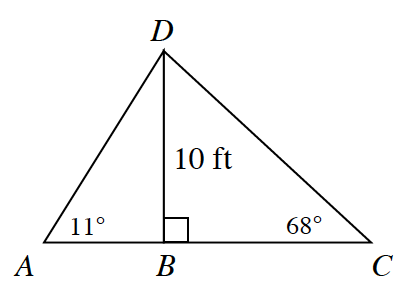### Home > INT2 > Chapter 2 > Lesson 2.1.4 > Problem2-44

2-44.

Examine the figure at right, which is not drawn to scale. Which is longer, $\overline{AB}$ or $\overline{BC}$? Explain your answer.

Calculate the measure of each angle created at vertex $D$.
Remember, in a given triangle, the longer side is opposite the larger angle.